# Divide

Divide substance 110 cm long to two parts so that it first part is 10 cm longer than the second part and one part will be 10 times longer than the second portion. How long the parts will be?

Result

a =  60 cm
b =  50 cm

#### Solution:

a+b=110
a = 10+b

a+b = 110
a-b = 10

a = 60
b = 50

Calculated by our linear equations calculator.

Leave us a comment of example and its solution (i.e. if it is still somewhat unclear...):

Showing 0 comments:Be the first to comment!#### To solve this example are needed these knowledge from mathematics:

Do you have a linear equation or system of equations and looking for its solution? Or do you have quadratic equation? Do you want to convert length units?

## Next similar examples:

1. Dividing by five and tenNumber 5040 divide by the number 5 and by number 10: a = 5040: 5 b = 5040: 10
2. Wire cut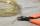A wire of length 7 m was cut into equal lengths using 4 cuts. How long is each piece?
3. FedorFedor stood in the small pool. Above the surface was a part of his body that was twice as long as part of his body underwater. What height does Fedor have, if his body part below the surface is 5 dm long?
4. Salary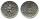Mr. Vesely got for work 874 CZK (Czech Republic Koruna). Mr. Jaros got twice less than Mr. Vesely. How much CZK got Mr. Jaros?
5. Bakers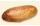Baker Martin baked 5 times more cakes than Dennis. How many cakes baked Dennis if Martin bake 25 cakes?
6. Math classificationIn 3A class are 27 students. One-third got a B in math and the rest got A. How many students received a B in math?
7. The resultHow many times I decrease the number 1632 to get the result 24?
8. DoctorsIn the city operates 171 doctors. The city has 128934 citizens. How many citizens are per one doctor?
9. Trains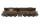Daily passes the same track section 8 pairs of trains with average 2400 passagers. How many people average travel in one train?
10. Unknown number xyzFind the number that its triple is 24. Solve by equation.
11. Forest nurseryIn the forest nursery after winter, they found that 1/10 stems died out of them. For them, they land 193 new spruces. How many spruces are in the forest nursery?
12. Class alphabet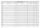All 29 pupils in the class are written in a class by alphabet. The number of pupils enrolled before Paul is three times higher than the number of pupils behind him. Calculate how many pupils are enrolled after Paul.
13. Jan and DanJan and Dan had the same money. Jan bought 5 workbooks and left him 15 CZK. Dan 6 and left him nothing. How much money have in total?
14. Eq1Solve equation: 4(a-3)=3(2a-5)
15. If-then equationIf 5x - 17 = -x + 7, then x =
16. Hotel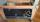The rooms in the mountain hotel are double and triple. Double rooms are 25 and triple are 17 more. How many rooms are there in this hotel?
17. Addition of Roman numbersAdded together and write as decimal number: LXVII + MLXIV